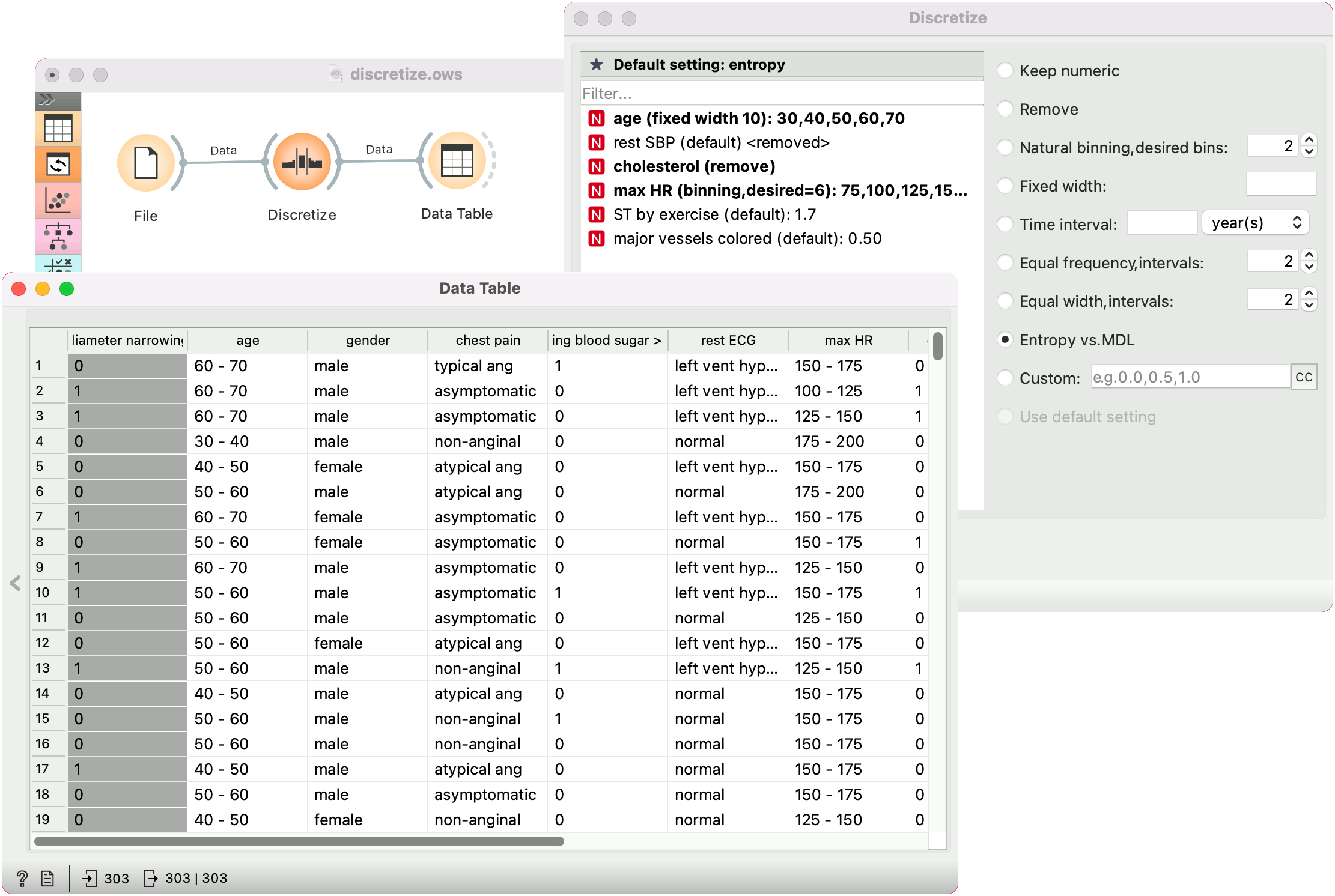# Discretize

Discretizes continuous attributes from an input dataset.

Inputs

• Data: input dataset

Outputs

• Data: dataset with discretized values

The Discretize widget discretizes continuous attributes with a selected method.1. The basic version of the widget is rather simple. It allows choosing between three different discretizations.
• Entropy-MDL, invented by Fayyad and Irani is a top-down discretization, which recursively splits the attribute at a cut maximizing information gain, until the gain is lower than the minimal description length of the cut. This discretization can result in an arbitrary number of intervals, including a single interval, in which case the attribute is discarded as useless (removed).
• Equal-frequency splits the attribute into a given number of intervals, so that they each contain approximately the same number of instances.
• Equal-width evenly splits the range between the smallest and the largest observed value. The Number of intervals can be set manually.
• The widget can also be set to leave the attributes continuous or to remove them.
2. To treat attributes individually, go to Individual Attribute Settings. They show a specific discretization of each attribute and allow changes. First, the top left list shows the cut-off points for each attribute. In the snapshot, we used the entropy-MDL discretization, which determines the optimal number of intervals automatically; we can see it discretized the age into seven intervals with cut-offs at 21.50, 23.50, 27.50, 35.50, 43.50, 54.50 and 61.50, respectively, while the capital-gain got split into many intervals with several cut-offs. The final weight (fnlwgt), for instance, was left with a single interval and thus removed. On the right, we can select a specific discretization method for each attribute. Attribute “fnlwgt” would be removed by the MDL-based discretization, so to prevent its removal, we select the attribute and choose, for instance, Equal-frequency discretization. We could also choose to leave the attribute continuous.
3. Produce a report.
4. Tick Apply automatically for the widget to automatically commit changes. Alternatively, press Apply.

## Example

In the schema below, we show the Iris dataset with continuous attributes (as in the original data file) and with discretized attributes.